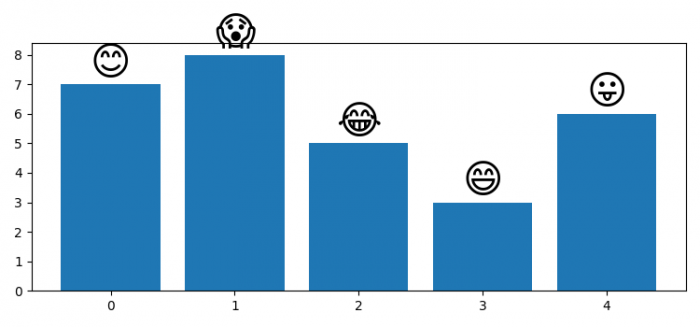# How to plot an emoji as a label for a bar in Matplotlib?

We can use annotate() to place an emoji at the top of a bar.

## Steps

• Set the figure size and adjust the padding between and around the subplots.
• Make a list of frequencies and labels conatining emojis.
• Create a new figure or activate an existing figure using figure() method.
• Plot bars using bar() method.
• Use annotate() method to place emojis as a label
• To display the figure, use show() method.

## Example

import numpy as np
from matplotlib import pyplot as plt
plt.rcParams["figure.figsize"] = [7.50, 3.50]
plt.rcParams["figure.autolayout"] = True
freqs = [7, 8, 5, 3, 6]
labels = ['😊', '😲', '😂', '😃', '😛']
plt.figure()
p1 = plt.bar(np.arange(len(labels)), freqs)
for rect1, label in zip(p1, labels):
height = rect1.get_height()
plt.annotate(
label,
(rect1.get_x() + rect1.get_width()/2, height+.05),
ha="center",
va="bottom",
fontsize=30
)
plt.show()

## Output